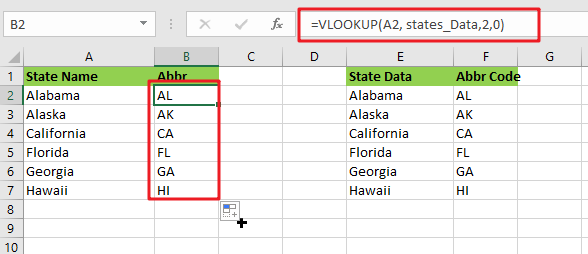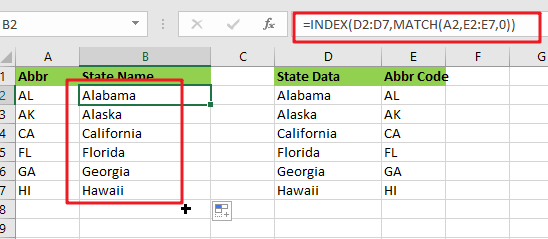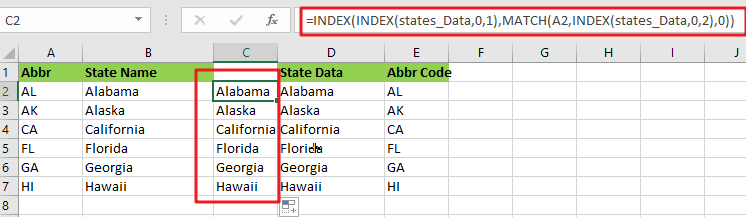# Convert State Names To Abbreviations

Assume that you got a task to convert the full state’s name into the abbreviations in MS Excel, and for doing this task, you might choose to do it manually, which is an acceptable way only if you don’t have any other way to do it!

But fortunately, there is a way to convert a state’s name into abbreviations, and after reading this article carefully, you would get to know about that way by which you can do this task in a few seconds.

So let’s get straight into it,## General Formula

The Formula to convert state names to the abbreviations in a matter of seconds is mentioned as follows:

`=VLOOKUP(States_Name,states_Data,2,0)`

## Explanation of Syntax

Before getting knowledge about how to use this Formula to get the work done, we first need to understand how each syntax of the Formula contributes to making this Formula worthful:

• `VLOOKUP`: The VLOOKUP function retrieves data from a range or table row by row.
• `Parenthesis` (): The primary function of this symbol is to group the elements.

## Let’s See How This Formula Works

A simple formula based on VLOOKUP may be used to convert complete state names to their two-letter abbreviation. The Formula in B2 in the example is:

`=VLOOKUP(A2, states_Data,2,0)`Where “ states_Data ” refers to the designated E2:F7 range:

This means that this method is based on a database with columns for the full state name and the 2-letter abbreviation. Because we’re using VLOOKUP, we need the whole name in the first column. The table has been dubbed “states_Data” for clarity’s sake.

VLOOKUP is set to retrieve the lookup value from column A. The table array represents the designated range “ states_Data,” The column index is 2 (to extract the abbreviation from the second column). The final option, range lookup, has been set to zero (FALSE).

VLOOKUP finds the matching entry in the “states_Data” database and returns the 2-letter abbreviation.

## Lookup In Reverse

What if you have an abbreviation of the states and want to search up the complete state name in the sample lookup table? You’ll need to use INDEX and MATCH instead in such a situation. With a lookup value in A2, this Formula will yield the whole state name as shown in the lookup table:

`=INDEX(D2:D7,MATCH(A2,E2:E7,0))`You may use this version to convert a 2-letter abbreviation to a complete state name if you wish to utilize the same-named range “ states_Data.”

`=INDEX(INDEX(states_Data,0,1),MATCH(A1,INDEX(states_Data,0,2),0))`By specifying a row number of zero, we may utilize INDEX to return whole columns. This is an interesting and helpful aspect of the INDEX function: if you specify zero for the row, you receive complete column.

You may convert abbreviations to complete state names using the INDEX and MATCH methods. The INDEX and MATCH functions can help you out to retrieve the complete state names depending on the abbreviations.

### Related Functions

• Excel VLOOKUP function
The Excel VLOOKUP function lookup a value in the first column of the table and return the value in the same row based on index_num position.The syntax of the VLOOKUP function is as below:= VLOOKUP (lookup_value, table_array, column_index_num,[range_lookup])….
• Excel INDEX function
The Excel INDEX function returns a value from a table based on the index (row number and column number)The INDEX function is a build-in function in Microsoft Excel and it is categorized as a Lookup and Reference Function.The syntax of the INDEX function is as below:= INDEX (array, row_num,[column_num])…
• Excel MATCH  function
The Excel MATCH function search a value in an array and returns the position of that item.The MATCH function is a build-in function in Microsoft Excel and it is categorized as a Lookup and Reference Function.The syntax of the MATCH function is as below:= MATCH  (lookup_value, lookup_array, [match_type])….
Related Posts

Build Hyperlink With VLOOKUP in Excel

You might have come across a task in which you were assigned to build hyperlinks, which seems very easy, and if you are new to excel or don't have enough experience with it, then you might wonder about doing this ...

Calculate Total Cost with Excel VLOOKUP Function

In today's article we will show you how to calculate the total cost for a given weight using the Excel VLOOKUP function. This function will help us to find the appropriate unit price for that weight and then we can ...

Basic Price Discount Calculation with Excel VLOOKUP Function

We often encounter product discounts in our shopping. Depending on the level of spending, the mall will offer different percentages of discounts. Usually, the more you spend, the bigger the discount, while the less you spend, the smaller the discount. ...

Calculate Grades With VLookup in Excel

Why Should You Calculate Grades With VLookup Excel? If you're looking for a simple way to find out a student's grades, VLOOKUP Excel can do the trick. This function uses a lookup table to find the values and sort them ...

Find and Replace Multiple Values

This post will guide you how to find and replace multiple values at once with VBA macro or using formula  in Excel. How do I make multiple find and replace in Excel. Suppose that you have a few cells containing ...

Excel XLOOKUP Function

Excel XLOOKUP Function was added into Excel as a beta feature in August 2019 and is now accessible exclusively in Microsoft 365. (as of July 2021). However, if you fall into this category and often deal with big sets of ...

VLOOKUP Formula | Faster Trick with 2 VLOOKUPS

This post will guide you how to use 2 VLOOKUPS function to looking up data entries from a given range of cells in Microsoft Excel. VLOOKUP with 2 lookups can be faster than a single VLOOKUP in certain scenarios. The ...

VBA Macro For VLOOKUP From Another Sheet

In the previous post, you should know that how to fix or remove the #N/A error when using VLOOKUP formula to lookup value from another sheet. And this post will show you how to use VBA code to vlookup data ...

VLOOKUP From Another Sheet Not Working

In the previous post, you should know that how to fix or remove the #N/A error when using VLOOKUP formula to lookup value from another sheet. And this post will show you reasons why your VLOOKUP formula is not working ...

Fix #N/A Error For VLOOKUP From Another Sheet

This post will show you how to fix the #N/A error why it occurs when you extract values from another sheet using VLOOKUP function in Excel 2016,2013,2010 or other Excel versions. How can you correct a #N/A error in VLOOKUP ...

Sidebar